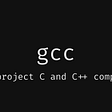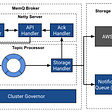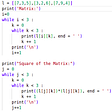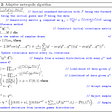# Circular Convolution using python

`# 1 ) input x(n) and h(n)# 2 ) For circular convolution we need N*N matrix so initializing empty matrix with np.zeros((shape))# 3 )Copying the x(n) into the first row of the matrix# 4 ) Run a for loop from 1 st row to Nth row.# 5 )In each iteration circularly shift the row vector# 6 )Take transpose of the matrix to get regular form as in circular convolution matrix# 7 ) Make sure that x(n) and h(n) are of same length .# 8 ) Multiply generated matrix with matrix h(n)`
`import numpy as np# circular shift operation# [1 2 3 4] = [4 1 2 3]def shifter(matrix):    last = matrix[len(matrix)-1]    x = len(matrix)    result =  * x    for i in range(1,len(matrix)):    result[i] = matrix[i-1]    result = last    return result#  finding circular convolutiondef findCircularConvolution(x,h,n,m):    primary_matrix = np.zeros((max(n,m),max(n,m)))    for i in range(0,len(primary_matrix)):    primary_matrix[i] = x[i]    for i in range(1,max(n,m)):        primary_matrix[i] = shifter(primary_matrix[i-1])        ultimate_matrix = np.transpose(primary_matrix)    difference_in_length = abs(n-m)    for i in range(m,m+difference_in_length):         h.append(0)   resultant = np.dot(ultimate_matrix,h)   return resultantx = [int(x) for x in input('Enter the x(n) -> ').split()]h = [int(x) for x in input('Enter the h(n) -> ').split()]circular_convolution_result = findCircularConvolution(x,h,len(x),len(h))print(circular_convolution_result)`

--

--

--

## More from Nilay Paul

Love podcasts or audiobooks? Learn on the go with our new app.

## How to choose home screen in Flutter## What happens when you type gcc main.c## iOS Developers Ship 29% Fewer Apps in 2017, the First Ever Decline + More Trends to Watch In 2018## Developing in Dagster## Whatsapp bringing new exciting features## Introduction to Ruby Rack Application## MemQ: An efficient, scalable cloud native PubSub system## Matrix in Python## Adaptive metropolis algorithm Python code## Python: Input and Output